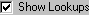# Vensim Help

 Navigation: Reference Guide > Functions Lookups

In addition to the predefined functions, you can specify an arbitrary nonlinear relationship with a Lookup.  Put simply, a Lookup is a list of numbers representing an x axis and a y axis.  The inputs to the Lookup are positioned relative to the x axis, and the output is read from the y axis.  You use Lookups to create your own specialized functions.

Lookups are also referred to as "Lookup Functions,"  "Tables," "Lookup Tables," "Table Functions," and sometimes "Graphical Functions."

Lookups can be declared as x,y pairs or by specifying the x-axis followed by the y-axis.  The format for declaring a Lookup is:

LOOKUP NAME([(Xmin, Ymin)-(Xmax, Ymax),(Xref1, Yref1),(Xref2,Yref2),...(Xrefn,Yrefn)]

(X1, Y1), (X2,Y2), . . .(Xn, Yn) )

 ~ Units ~ Description  |

or:

LOOKUP NAME( X1, X2, X3,....Xn, Y1, Y2, Y3,....Yn)

 ~ Units ~ Description |

In the second case, the number of entries for X needs to match the number of entries for Y.  For all Lookups, a minimum of two values must be specified for both the x and y axis.

Lookups are most commonly defined using the Graph Lookup Editor:The Graph Lookup Editor allows you to enter x,y pairs, or to trace the shape of the Lookup using the mouse.  The Graph Lookup Editor is described in detail in Editing Lookups.

When you use the Graph Lookup Editor, it will put additional information on scaling in the lookup definition as in:

 effect of energy function([(0,0)-(4,2)],(0,0),(0.501742,0.596491),
 (1,1),(1.44948,1.34211),(2,1.6),(2.99652,1.7807),(4,1.8))

The range shown in square brackets [(0,0)-(4,2)] is used by the Graph Lookup Editor to determine the scaling.  It can also be followed by optional reference point information.  There is no need to enter a scale or reference point information when typing in Lookup values.